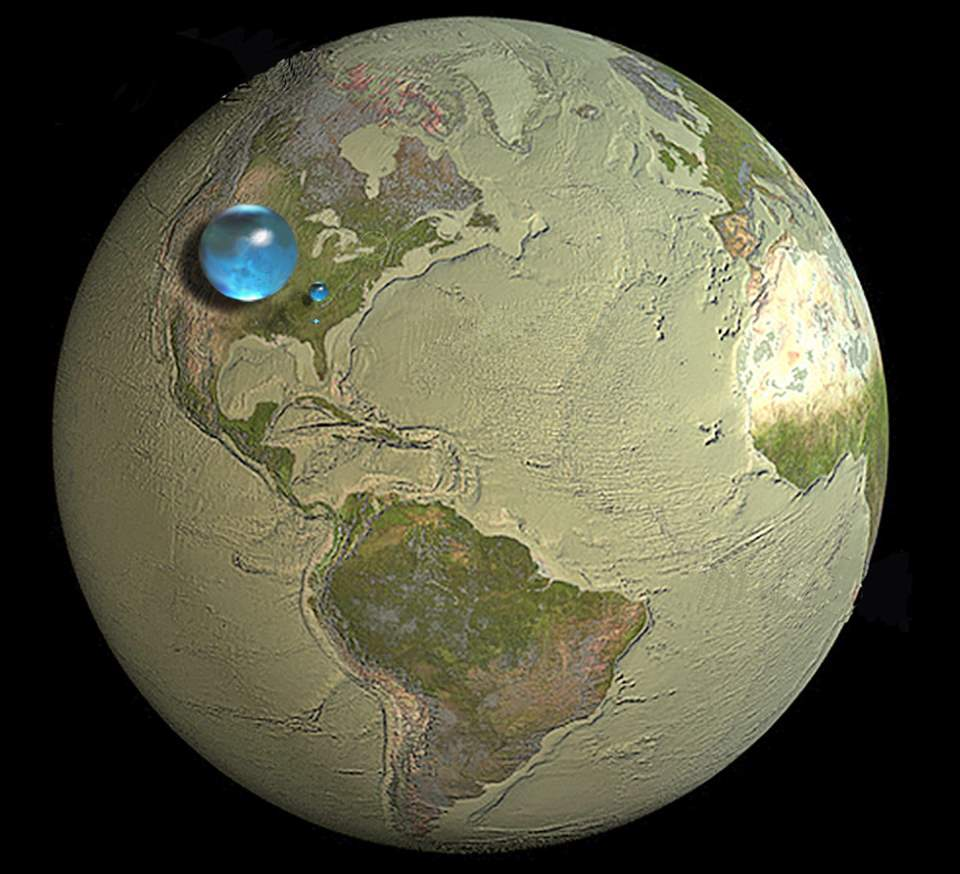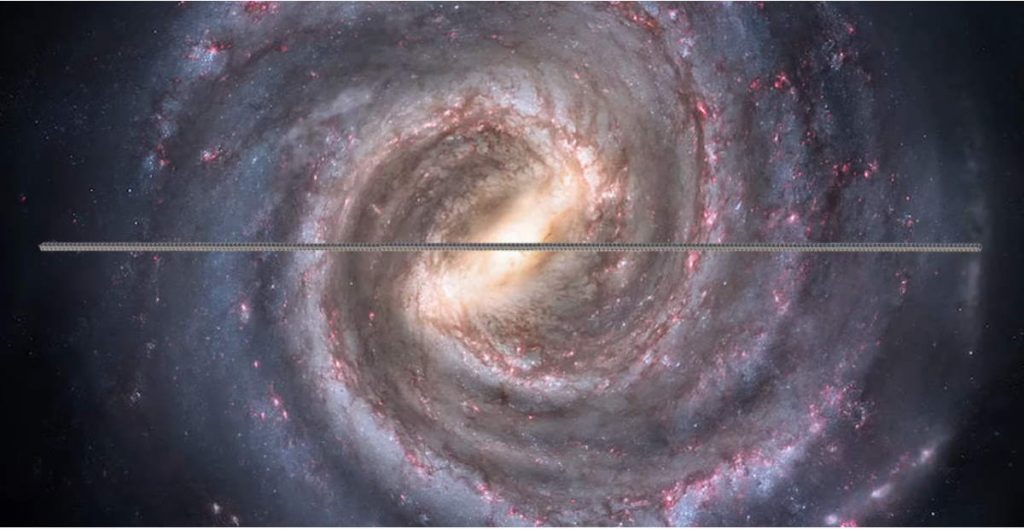“How long is the Earth” is a weird question – but what if you cut it up into Minecraft-sized 1-meter cubes and lay them in a line through space, how far would the Earth stretch? It is a thought experiment and in the video published by the astrophysicist (and a famous poker player!) Liv Boeree below, we can get the answer and it is mind-blowing: the Earth would stretch across the entire Milky Way (more than 100,000 light-years!) if its volume is laid out in a line of 1-meter cubes.

Boree says “volume is seriously underrated” and it’s true.

For example, some people can’t get the illustration below – which shows the volume of water on Earth. They think “70 percent of Earth is covered with oceans, the volume of water (the size of the water spheres below) cannot be that small compared to the volume of Earth”. But they are simply wrong – they simply cannot understand the volume and the math behind its calculation.All Earth’s water, liquid fresh water, and water in lakes and rivers Spheres showing: 1. All water (sphere over the western U.S., 860 miles/1384.04 km in diameter) 2. Fresh liquid water in the ground, lakes, swamps, and rivers (sphere over Kentucky, 169.5 miles/272.78 km in diameter), and 3. Fresh-water lakes and rivers (sphere over Georgia, 34.9 miles/56.17 km in diameter). Credit: Howard Perlman, USGS; globe illustration by Jack Cook, Woods Hole Oceanographic Institution (©); Adam Nieman.

The area is measured in square units. Volume is measured in cubic units such as cubic inches or cubic centimeters.

For example, let’s think about a 1-meter square. Its area is 1×1 = 1 square meter, and if we put a cube on it, its volume would be 1x1x1 = 1 cubic meter.

Now let’s double the square’s sides. Now its area is 2×2 = 4 square meters, but a cube on top of it would have a volume of 2x2x2 = 8 cubic meters.

If it was 10 meters x 10 meters square, then we get 100 square meters, but a cube’s volume would be 10x10x10 = 1000 cubic meters!

You can continue forever – for a side of 100 meters, the area would be 10,000 square meters while the volume would be a whopping 1 million cubic meters. That’s 1 million tonnes of water if you fill that cube with water!

## Calculations behind the “How LONG is the Earth” video

Earth’s volume is approx. 10^21 cubic meters. The diameter of the Milky Way galaxy is estimated at around 100,000 light-years, which is approximately = 10^21 meters. Hence, the earth disassembled and rearranged into a single line of 1-meter sided cubes stretches across our galaxy.How long is the Earth? the Earth would stretch across the entire Milky Way (more than 100,000 light-years!) if its volume is laid out in a line of 1-meter cubes.

### Observable universe length Earth

Assuming the observable universe of approximately 90 billion light-years in diameter = 8.5×10^26 meters (D) and Volume V of Earth of 10^21 cubic meters, then the size of cubes required is:

X = √(V/D) = 1.12 mm

In Big Bang cosmology, the observable universe is what, in theory, can be seen from Earth.

The observable universe is a spherical volume (a ball) centered on the observer, regardless of the shape of the universe as a whole. Every place in the universe has its own observable universe, which may or may not overlap with the one centered on Earth. There are at least 2 trillion galaxies in the observable universe.

Beyond that (beyond the observable universe), the light from objects has not had enough time to reach the Solar System and Earth since the beginning of the cosmological expansion. That’s why the sky is dark at night.